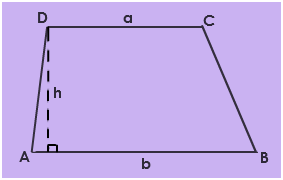# Area of a Trapezoid Formula

A trapezoid is described as a 2-dimensional geometric figure which has four sides and at least one set of opposite sides are parallel. The parallel sides are called the bases, while the other sides are called the legs. There are different types of trapezoids: isosceles trapezoid, right trapezoid, scalene trapezoid. A trapezoid with the two non-parallel sides of the same length is called an isosceles trapezoid. A right trapezoid is a trapezoid that has at least two right angles. A right isosceles trapezoid is a trapezoid that is simultaneously a right trapezoid and an isosceles trapezoid. In Euclidean geometry, such trapezoids are automatically rectangles.Area of a Trapezoid = A =
$$\begin{array}{l}\frac{1}{2}\end{array}$$
$$\begin{array}{l}\times\end{array}$$
h
$$\begin{array}{l}\times\end{array}$$
(a + b)
Where:
h = height (Note – This is the perpendicular height, not the length of the legs.)
a = the short base
b = the long base

### Solved Example

Question 1: Find the area of a trapezoid whose bases are 17 cm and 12 cm and height is 7 cm.
Solution:
Given,
a = 12 cm
b = 17 cm
h = 7 cm
Area of a trapezoid
=
$$\begin{array}{l}\frac{1}{2}\end{array}$$

h(a + b)
=
$$\begin{array}{l}\frac{1}{2}\end{array}$$
7
(12 + 17) cm2
=
$$\begin{array}{l}\frac{1}{2}\end{array}$$
7
(29) cm2
=
$$\begin{array}{l}\frac{1}{2}\end{array}$$
* 203 cm2
= 101.5 cm2

 More topics in Area of a Trapezoid Formula Isosceles Trapezoid Formula